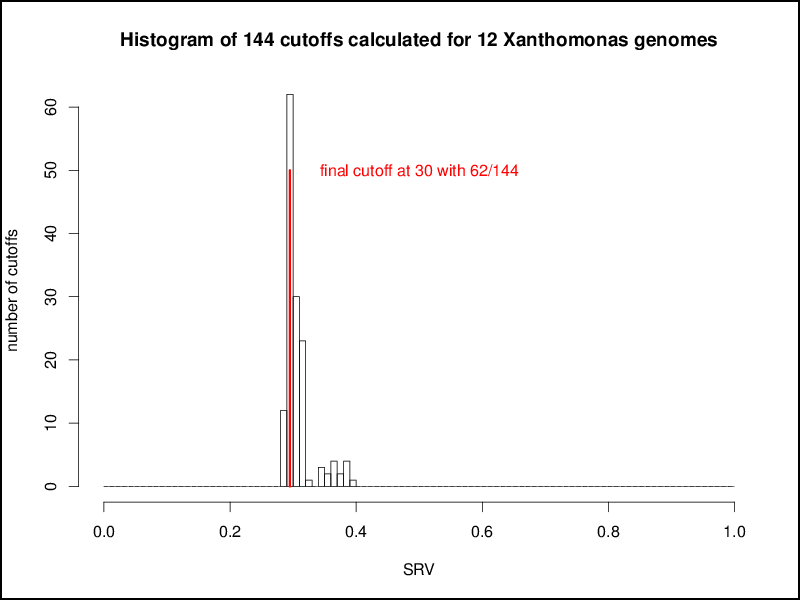# Orthology calcuation

For orthology estimation EDGAR uses a generic orthology threshold calculated from the similarity staitistics of the compared genomes. Here you can find an in-depth description of the orthology calculation method implemented in EDGAR.

For the high-throughput computation of comparative data it is crucial to rely on a generic orthology criterion consistent within the analyzed genome set. For this purpose, EDGAR deploys the so called BLAST Score Ratio Values (SRVs) suggested by Lerat et al. (2003).

Instead of using the absolute bit scores provided by the BLAST algorithm, the SRV method uses a normalization approach by relating all bit scores of a protein to the maximum bit score that can be achieved by this protein sequence. The maximum bit score is defined as the BLAST bit score of an alignment of the sequence against itself, as such a BLAST hit always has 100% identity over 100% of the query sequence length and thus gives the maximum bit score possible. So, a SRV is defined as the ratio (Observed score=Maximum score), thus giving a value in the range [0; 1].

Finally, Lerat et al. observed that the distribution of bit scores from a all-against-all comparison of the genes of two related genomes shows a bimodal pattern. The first peak at low similarity values was constant among comparisons, thus most probably representing random matches, while the second peak at higher similarities represents true homologous sequences.

To remove all nonspecific hits, a cutoff value has to be identied that defines the boundaries of the first peak and thus the set of random hits that should be removed. Lerat et al. analyzed the class of Gamma-Proteobacteria and chose a fix SRV threshold of 30% for orthology estimation based on manual inspection of the bimodal SRV distribution. For EDGAR we wanted to develop an automatic, unsupervised estimation to identify an appropriate threshold.

To find a cutoff for the comparison of two genomes we use a statistical approach based on the beta distribution. The number of BLAST hits with a given SRV is summed up and represented in a histogram for all SRV values in the range [0; 100]. As the beta distribution is defined in an interval [0; 1] the SRV histogram is normalized to this interval. Due to the fact that we want to remove the first peak, we only use all values in the left part of the SRV histogram with a normalized SRV < 0.4 and calculate a beta distribution from the mean and standard deviation of the observed SRVs within this interval. The density function of this beta distribution is calculated, and the 97% quantile of this density function is taken as cutoff that defines the end of the first peak. The value of 97% was chosen based on manual inspection of hundreds of SRV distributions. The typically used values of 95% (single standard deviation) or 99% (double standard deviation) were discarded as 95% gave cutoff values that seemed slightly too low and 99% gave values slightly too high. A normalized SRV distribution with the fitted beta distribution density function is shown in the following figure:This procedure is repeated for all possible combinations of genomes, resulting in n2 combinations for a set of n genomes. These n2 cutoffs could be used as threshold for a multi genome comparison by using a distinct cutoff for every comparison of two genomes. This would result in a higher accuracy as for every pairwise comparison a tailored cutoff would be used, but at the same time the basis of comparison for the overall calculations would be lost, e.g, for a comparison of a number of genomes the singletons of each genome would be calculated with different cutoffs. Hence, as distinct cutoffs would give hardly comparable results, the aim of the EDGAR threshold estimation mechanism was to calculate one master cutoff for all genome comparisons. To obtain this the n2 cutoffs are plotted in a second histogram, which in most cases shows a normal distribution. To get a comparable general threshold for all subsequent calculations, the peak of this histogram is determined and used as the master cutoff for the BBH computation between the compared genomes:Based on the master cutoff calculated as described above EDGAR condisers two genes to be orthologous to each other if:

1. The have reciprocal best BLAST hits (BBHs)
2. The SRV values of both single BBHs is above the calculated cutoff.

The orthology cutoff generated by this approach is quite strict, as all low quality BLAST hits are filtered out. In this way it supports the desired high specificity of the orthology estimation. In an example EDGAR project with 42 organisms from the genus Erwinia, the calculated cutoff is 31. Using an initial evalue cutoff of 1e−5 about 40 million BLAST results are parsed, of which only ∼7.3 million or 18.25% pass the SRV filter. The mean percent identity of all hits of 73.5 (median 79.0) and the mean evalue of 6.6e−9 (median 6.0e−103 ) confirm the strictness of the filter. As a consequence of that strict threshold, orthologs found by EDGAR, especially when conserved among numerous genomes like the core genes, could be considered real orthologs, but some potential orthologs might be lost.